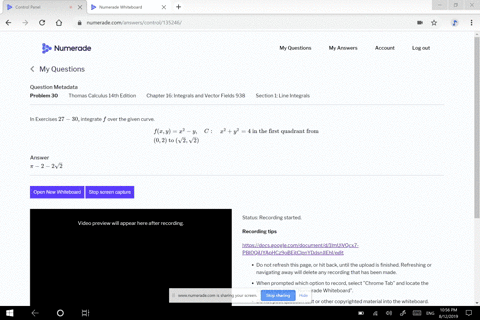🎉 The Study-to-Win Winning Ticket number has been announced! Go to your Tickets dashboard to see if you won! 🎉View Winning Ticket### In Exercises $27 - 30 ,$ integrate $f$ over the g…

01:17University of Central Arkansas
Problem 29

# In Exercises $27 - 30$ , integrate $f$ over the given curve.$$\begin{array} { l } { f ( x , y ) = x + y , \quad C : \quad x ^ { 2 } + y ^ { 2 } = 4 \text { in the first quadrant from } } \\ { ( 2,0 ) \text { to } ( 0,2 ) } \end{array}$$

## Discussion

You must be signed in to discuss.

## Video Transcript

Okay. What we want to do is we want to integrate. Um, the function f over the giving curve. Okay, so our function is defined to be, um X plus y, and the curve is to find to be X squared, plus y squared equal to four in the first quadrant. So it's going to go from to zero two 02 Okay, So what we need to do is to, first of all, to find, um or er tea. So we're gonna change this. This is a circle with a radius of two. And so when we change it into its parametric form, we know the X value is to co sign of tea I and the why is, um too sign of tea j. And so now we need Thio. Um, Then we also need to talk about our tea value and in the first quadrant is gonna go from zero to pi over, too. And so if I take the derivative of r of T, that's gonna give me V of tea. And so that becomes negative to sign of tea. I plus to co sign of tea. Okay. And so the magnitude of e of t is equal to the square root of negative to sign of t squared plus a to co sign of tea and we're going to square that. And so this just reduces down to two because we end up with the sine squared Prosecco Scott co sign squared, which is a one Hey, two squares That gives me the four. So the square root of forced to and so D D s is equal to two TT And so we set up our integral So the integral role we have that too outside we're going from zero to pi over two and we're going from X, which is to co sign of ti umm and plus a Y, which is to sign of tea and d t. And so this just becomes a two time's a to sign of tea minus a to co sign of tea. And we're evaluating it from at Pi over two and then again at zero. And so when we do ah, we kid two times two, um, minus zero minus, um evaluate visit at zero. And then this would be a minus two. And so I end up getting a four inside the brackets times a to which gives me the eight